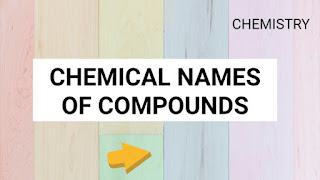# Chemical Formulas of compounds -Chemistry

Chemical Formulas of Compounds of Chemistry:Chemical Formulas of Compounds

1. What is the Chemical formula of Methanol ?
Ans. CH3OH.

2. What is the Chemical formula of Ethane ?
Ans. C2H6.

3. What is the Formula of Gold ?
Ans. Au.

4. What is the Chemical formula of Iodine ?
Ans. I.

5. What is the Chemical formula of Benzene ?
Ans. C6H6.

6. What is the Chemical formula Butane ?
Ans. C4H10.

7. What is the Chemical formula of Methane ?
Ans. CH4.

8. Chemical formula of Propane:
Ans. C3H8.

9. Chemical Formula of Sulphuric acid :
Ans. H2SO4.

10. What is the Chemical Formula of Carbon Dioxide :
Ans. CO2.

11. Chemical Formula of Copper :
Ans. Cu.

12. Chemical Formula of Water :
Ans. H2O.

13. Chemical Formula of alcohol:
Ans. C2H6O.

14. What is the Chemical Formula of Glucose ?
Ans. C6H12O6.

15. What is the Chemical Formula of Sodium:
Ans. Na.

16. What is the Chemical formula of Aspirin ?
Ans. C9H8O4.

17. Chemical formula for Hydrochloric Acid :
Ans. HCl.

18. Chemical formula For tin:
Ans. Sn.

***                **